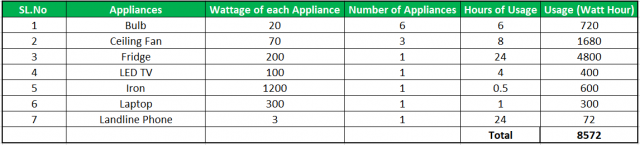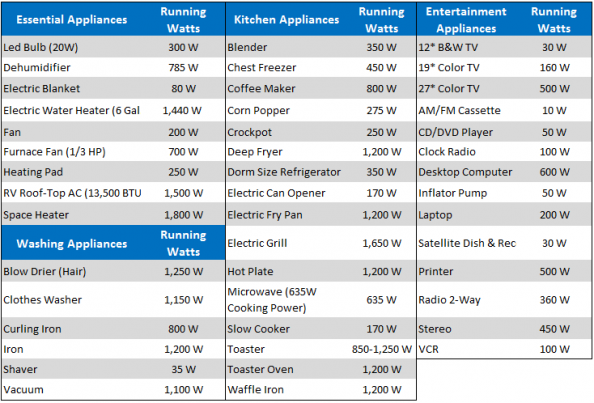# Solar System Size Calculation

Size Calculation of a solar system is the topmost priority before deciding the capacity of the system. In this blog, we are going to discuss the size of the major components like solar panels, solar inverter and solar batteries in an off-grid system. For Solar system size, we need to check our energy requirements in terms of kWh(kilowatt-hour) a unit of consumption and then we will calculate the system capacity and then all components size can be calculated. There are many methods for calculating energy requirement but we are going to discuss two major and easy ways for calculating.

## Method 1:

This method is for those who have permanent electricity connection in their house/property and if you are getting electricity bills from the distribution company. Search in the monthly lastest electricity bill for the units of consumption (consumption Units). The value determines how much energy you have consumed from the electricity board in the particular month. Divide the units of consumption by 120. The value comes after dividing defines the size of the system. If the value comes 4 then you have to install 4 kwatt of solar power system so it will generate the required energy and you will not get the electricity bills.

If you don’t have an electricity bill, then you have to calculate how much each appliance are consuming energy per month. This type of calculation will be approximate because you can’t calculate how much you are consuming or going to consume energy from a particular appliance. Please follow the below tables for the calculation:

• Wattage of each appliance: Every appliance have their own wattage value,
• Usage (Watt-Hour) = Wattage of each appliance * No. of appliance * Hours of usage
• Sum all the watt- hour of an individual appliance.As we can see in the table, 8572 watt-hour is the consumption. We have to convert it to kilo-watt hour by dividing 8572 by 1000. 8.57 units are the consumption per day. Refer the below table for more info on other appliances that you might use.Source: Generatorist.com

From any of the methods shown above we can calculate our energy requirements. For sizing the components let us take an example.

• Monthly usage = 480 units
• Daily usage= 480/30= 16 units
• Night Usage (50% of the total day usage)= 8 units

### Solar Panels:

Solar Panels is one of the major components of solar power system which generates energy when it comes with the contact of the sun.Solar panels come in different sizes and you can choose them according to the availability and your personal preference.

For standard calculation, we consider that solar panels of 1 kwatt capacity generate 4 units per day and 120 units (considering 30 days month). So to get 480 units of generation from solar we need 480/120 equals to 4 kwatt of solar panels. If you use 325 wp of solar panel capacity you have to take 4000 watt/ 325 wp equals 12-13 number of solar panels.

### Solar Inverter

Solar inverter is the brain of the system and its work is to convert the DC energy coming from solar panels to AC energy and supply the energy to our appliances. Solar Inverter of 4 kwatt capacity is required as per the current example.

### Solar Battery:

Solar generation happens only in day time when sun is available. For the time when solar energy is not present, we need to store it in the batteries. Now the biggest task is to calculate how much batteries and what capacity of the battery is required.

• Number of batteries:  Depends on inverter specifications. In the datasheet/technical specification sheet of the inverter, it is always mentioned as battery bank voltage as 48 volt / 96 volt / 120 volt depends upon the size of the inverter. For a 4 kwatt inverter battery bank voltage is 48v. Each battery works on 12 volt (90% of cases). So let us consider battery voltage as 12 volts and battery bank voltage is 48 volts. The number of batteries required will be 48v/12v equals 4. We have to use 4 number of batteries in series to make 48v battery system. For example, if the battery bank is 96v then 96v/12v equals to 8 batteries. So, 8 batteries are required in the system.
• Capacity of each Battery:
• Calculate(approx) your night consumption since battery power will be mostly used in the night.
• If we take 50 percent of the total day usage as night usage then 50% of  16 units (per day consumption as per our monthly usage of 480 units) equals 8 units.
• Battery bank should store 8 units to meet your night consumption.
• Battery works on DoD (Depth of Discharge) generally equal to 50% that means only 50% of energy stored in batteries are useful. So batteries need to store double of what is required equals to 8*2= 16 units (kWh)
• (16000 wh/48 volts)= 333 Ah.
• Summary: 4 number of 400 Ah battery is required to meet the requirements as 333 Ah battey is not available and the nearest is 400 Ah.

#### Summary:

1. Solar Panels: 325/330 wp solar panels 12 numbers.
2. Inverter: 1 number of size 4 kwatt.
3. Battery: 4 numbers of 400 Ah

#### Final Say:

In this way, we can calculate our energy requirements and we can size different components of the system. One of the best solar companies like SolarClue provides a comprehensive range of best solar systems. Its range of solar power system caters to the requirements of both domestic as well as commercial sectors. Buy solar components at industry leading prices from SolarClue, which is a noted supplier in the solar power industry. Solarclue is a leading solar installation company where people also can get a portable solar panel, solar inverter battery, and other essential components with proper strategy, planning.

### 9 thoughts on “Solar System Size Calculation”

•30/03/2020 at 3:19 am

Nice and simple article which can be easily understood. If you can also add the tentative cost of the equipment and if possible the installation charges, it would have been a complete article.

•30/03/2020 at 9:11 am

We made it simple to understand only the sizing part, becuase almost all the people gets confused in sizing. In our upcoming blogs we will tell the tentative cost of the on-Grid and an off-Grid System

•22/06/2020 at 7:19 am

Great article. Thanks for sharing it with us.

•24/06/2020 at 11:46 am

Hello,

•03/07/2020 at 6:12 am

How many cost of 4kw solor system with inverter and bettry

•03/07/2020 at 10:32 am

Hello,

It would be approximately RS. 3 Lakhs.

•23/02/2021 at 7:40 am

I’m really enjoying the design and layout
of your website. It’s a very easy on the eyes which makes it much more pleasant for me to come here and visit more often. Did
you hire out a developer to create your theme? Outstanding work!

•